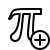# Symmetry analysis in fluid mechanicsLie groups, symmetry, and fluid mechanics

## Similarity and Transport Phenomena in Fluid Dynamics

Course in English (28 hr, 2 credits): The course is an introduction to Lie groups and symmetry analysis, with applications to conservation equations in fluid mechanics. The course aims to provide the student with mathematical and physical tools to deal with transport equations in continuum mechanics. Emphasis is given to similarity and travelling-wave solutions.

Room: CM 1 113. Time: 12h15-14h every Friday as of 23 September 2022.

It is highly recommended that students bring a laptop (with Mathematica or Matlab installed) for the exercises.

Course material
Examination
homework (20 Jan. 2023, 6 pm). Here is the Mathematica notebook for plotting phase portrait.
Bibliography
• Symmetry, similarity solutions
• Barenblatt, G.I., Scaling, Self-Similarity, and Intermediate Asymptotics, 386 pp., Cambridge University Press, Cambridge, 1996
• Barenblatt, G.I., Scaling, Cambridge University Press, Cambridge, 2003.
• Bluman, G.W., and S.C. Anco, Symmetry and Integration Methods for Differential Equations, Springer, New York, 2002.
• Cantwell, B.J., Introduction to Symmetry Analysis, Cambridge University Press, Cambridge, 2002.
• Dresner, L., Similarity Solutions of Nonlinear Partial Differential Equations, 123 pp., Pitman, Boston, 1983.
• Dresner, L., Applications of Lie’s Theory of Ordinary and Partial Differential Equations, Institute of Physics Publishing, Bristol, 1999.
• Fazio, R., A similarity approach to the numerical solution of free boundary problems, SIAM Review, 40, 616-635, 1998.
• Germain, P., Méthodes asymptotiques en mécanique, in Dynamique des Fluides, edited by R. Balian, and J.-L. Peube, pp. 1-148, Gordon and Breach Science Publishers, London, 1973.
• Gratton, J., Similarity and self similarity in fluid dynamics, Fundamentals of Cosmic Physics, 15, 1-106, 1991.
• Gratton, J., and C. Vigo, Self-similar gravity currents with variable inflow revisited: plane currents, Journal of Fluid Mechanics, 258, 77-104, 1994.
• Hydon, P., Symmetry Methods for Differential Equations — A Beginner’s Guide, Cambridge University Press, Cambridge, 2000.
• Holmes, P., J.L. Lumley, and G. Berkooz, Turbulence, coherent structures, dynamical systems and symmetry, Cambridge university press, 1998.
• Ibragimov, N.H., CRC Handbook of Lie Group Analysis of Differential Equations, CRC-Press, Bocan Rota, 1995.
• King, A.C., J. Billingham, and S.R. Otto, Differential Equations: Linear, Nonlinear, Ordinary, Partial, 541 pp., Cambridge University Press, Cambridge, 2003.
• Olver, P.J., Application of Lie Groups to Differential Equations, Springer, New York, 1993.
• Sachdev, P.L., Self-Similarity and Beyond, Chapman & Hall, Boca Raton, 2000.
• Sedov, L., Similarity and Dimensional Methods in Mechanics, CRC Press, Boca Raton, 1993.
• Zohuri, B., Dimensional Analysis and Self-Similarity Methods for Engineers and Scientists, Springer, Basel, 2015.
• Parabolic and hyperbolic differential equations
• Courant, R., and K.O. Friedrich, Supersonic Flow and Shock Waves, 455 pp., Intersciences Publishers, New York, 1948.
• Smoller, J., Shock waves and reaction-diffusion equations, 581 pp., Springer, New York, 1982.
• LeVeque, R.J., Finite Volume Methods for Hyperbolic Problems, Cambridge University Press, Cambridge, 2002.
• LeVeque, R.J., Numerical Methods for Conservation Laws, Birkhäuser, Basel, 1992.
• Ketcheson, D.I., R.J. LeVeque, and M. del Razo, Riemann Problems and Jupyter Solutions, Society for Industrial and Applied Mathematics, Philadelphia, 2020. The contents of this book are available online.